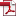### All Zero Block Detection of Transform Coefficients in HEVC

Vol. 6, No. 1, pp. 1-7, Mar. 202210.22662/IJEMR.2022.6.1.001, Full Text:Keywords: uniform quantization, HEVC, RDO quantization, all zero block, Hadamard transform

Abstract

During the transform and quantization process in HEVC, there are a lot of zero blocks. The coding time of transform and quantization can be considerably decreased by effectively detecting all zero blocks. This work proposes all uniform quantizer and RDO quantizer zero block detection techniques. The energy concentration property of DCT is used to suggest an improved transform coefficient estimate approach. Low frequency DCT coefficients are estimated using the Hadamard transform, while the maximum magnitude of high frequency DCT coefficients is estimated using a SATD-based technique. There will be an explicit threshold which can be used to compare the float quantization level of coefficients, and all zero blocks in the uniform quantizer may be effectively found. The stair-like thresholds for RDOQ are obtained through statistical analysis, which makes AZB identification easier. The experimental result shows that uniform quantizer’s coding time can be cut in half, and 40% coding time can be reduced for RDOQ.

Statistics